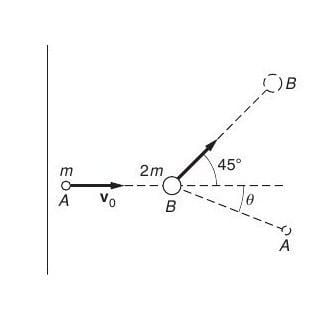# Particle collision and deflection angle

## Homework Statement

Particle A of mass m has initial velocity v0. After colliding with particle B of mass 2m initially at rest, the particles follow the paths shown in the sketch (see attachment). Find ##\theta##collisions

## The Attempt at a Solution

The momentum before and after collision is
##
\left\{
\begin{array}{}
\vec P_i = mv_0 \hat \imath \\
\vec P_f = (mv_0' \cos{\theta} + \sqrt{2}m v_1')\ \hat\imath + (-m \sin(\theta) v_0' +\sqrt{2}mv_1')\ \hat\jmath
\end{array}
\right.
##

By conservation of momentum, ##v_0'## and ##v_1'## can be expressed in terms of ##v_0## and ##\theta## :

##
\left\{
\begin{array}{}
v_0' = \frac{v_0}{\cos(\theta) +\sin(\theta)} \\
v_1' = \frac{v_0}{\sqrt{2}} \frac{\sin(\theta)}{\cos(\theta) +\sin(\theta)}
\end{array}
\right.
##

Now in terms of energy:

## K_f = \frac{1}{2}mv_0'^2 + m v_1'^2 = \frac{1+2t^2}{(1+t)^2} K_i##,

where ## t = \tan(\theta)##.
The ratio between energy lost in the collision and initial kinetic energy is:

## \alpha = \frac{Q}{K_i} = 1 - \frac{K_f}{K_i} = \frac{t(2-t)}{(1+t)^2} ##

which can be re-written :

## (\alpha+1) t^2 + 2(\alpha -1) t + \alpha = 0 ##

The roots are real numbers only if ##\alpha \le 1/3## which is the same as saying that no more than one third of initial energy can be lost in the collision. In that case:

## t = \frac{1-\alpha}{1+\alpha} \pm \frac{\sqrt{1-3\alpha}}{1+\alpha} =
\frac{K_i - Q}{K_i+Q} \pm \frac{\sqrt{K_i}\sqrt{(K_i - 3Q)}}{K_i+Q}##

The answer will be ##\theta = \arctan(t)##, but which root should we keep ?

Look at your diagram, which would be consistent with momentum conservation?

The diagram suggests that ##\theta## is smaller than 45 degrees. So we should have ##0<t<\frac{\sqrt{2}}{2}##.
For exemple, the '+' root is not acceptable for ##\alpha = 1/6##, so I think we have to keep the '-' root. Right?

Sorry, I made a mistake, ##\tan(45) = 1## and not ##\frac{\sqrt{2}}{2}##, but I reach to the same conclusion. Do you agree ?

haruspex
Homework Helper
Gold Member
The diagram suggests that θ is smaller than 45 degrees. So we should have 0<t<2√20
I wouldn't trust the diagram to show which angle is greater.
I don't understand your elaborate algebra dealing with fraction of energy lost. Don't you need to assume perfectly elastic in order to get an answer? It says particles, so maybe this is as in subatomic particles.

Hello,

Energy may or may not be conserved, the text does not say, that is why the result depends on Q.
The fraction of energy lost (##\alpha##) is a convenient way to link Q with the deflection angle ##\theta##.

My result is consistent with perfectly elastic collision: take Q = 0 and keep the '-' root. You'll find that the angle is 0. Conversely, that angle conserves kinetic energy.
However, the bigger particle would be at rest after the collision, which is in contradiction with the diagram. So the collision is inelastic.

Last edited:
haruspex
Homework Helper
Gold Member
My result is consistent with perfectly elastic collision: take Q = 0 and keep the '-' root. You'll find that the angle is 0. Conversely, that angle conserves kinetic energy.
It depends what you mean by a result. Your answer has in it an unknown, Q, which you introduced. The question as stated gives you no permission to do that.
Also, there's no way the angle can be 0. That would mean momentum is not conserved in the j direction.

wait...

For an elastic collision, ##\tan(\theta) = 0## or ##\tan(\theta) = 2##.
First option is impossible because momentum is not conserved.
Second option gives ##\sin(\theta) = 2\cos(\theta) ##.
That gives :
##K_f = \frac{K_i}{9}(\frac{1}{\cos^2(\theta)} + 4) ##

By conservation of energy, and if there is no mistake, ##\theta = \arccos(\frac{1}{\sqrt{5}}) ## which is approximately 63 degrees.

But why do you assume elastic collision, I don't understand

Last edited:
haruspex
Homework Helper
Gold Member
But why do you assume elastic collision, I don't understand
Because the question does not give enough information, so you have to ask what is it they forgot to tell you. Elastic collision is a possibility. Since it describes the objects as particles, perhaps you are intended to interpret these as subatomic particles. Perhaps there is more context you are aware of that might support that?

it makes sense, because of the lack of context, the answer is open to discussion. Now assume that the collision is inelastic and that Q joules are dissipated during the collision. You said earlier that you do not use the diagram to pick a root or another (the case of elastic collision proved you were right)
How would you do then ?

haruspex